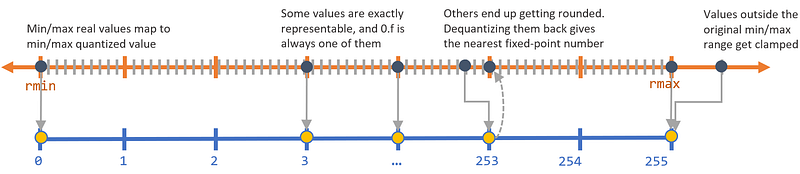# Quantization

the process of constraining an input from a continuous or otherwise large set of values (such as the real numbers) to a discrete set (such as the integers). An umbrella term that covers a lot of different techniques to store numbers and perform calculations on them in more compact formats than 32-bit floating point.For many deep learning problems, we’re finally getting to the “make it efficient” stage. We’d been stuck in the first two stages for many decades, where speed and efficiency weren’t nearly as important as getting things to work in the first place. So the question of how precise our calculations need to be — and whether we can manage with lower precision — wasn’t often asked. However, now that neural networks are good enough at many problems to be of production-grade or better, this question has arisen again. And the answers suggest we could do with low(er) precision, causing what may soon be a paradigm shift in mobile-optimized AI. Given this shift, this post explores the concept of quantized inference and how it works in TensorFlow Lite.

##### Quantization-aware model training

ensures that the forward pass matches precision for both training and inference. There are two aspects to this:

• Operator fusion at inference time are accurately modeled at training time.
• Quantization effects at inference are modeled at training time.

For efficient inference, TensorFlow combines batch normalization with the preceding convolutional and fully-connected layers prior to quantization by folding batch norm layers.

##### Post-training quantization

is a general technique to reduce the model size while also providing up to 3x lower latency with little degradation in model accuracy. Post-training quantization quantizes weights to 8-bits of precision from floating-point.• Pruning can remove lots of weights before doing quantization without hurting accuracy. Pruning can remove 67% for CONV layers, 90% for FC layers, verified across LeNet, AlexNet, VGGNet (shown in below paper), GoogLeNet, SqueezeNet, NeuralTalk (done recently after the paper) Learning both Weights and Connections for Efficient Neural Networks